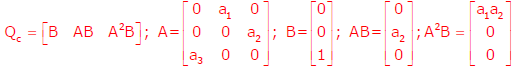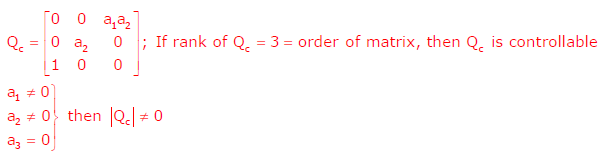# Signals and Systems - Online Test

Q1. A dual trace oscilloscope is set to operate in the Alternate mode. The control input of the multiplexer used in the y-circuit is fed with a signal having a frequency equal to
Explaination / Solution:
No Explaination.

Q2. The fourier series expansionof the periodic signal shown below will contain the following nonzero terms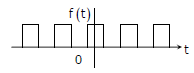Explaination / Solution:

⇒ it satisfies the half wave symmetry, so that it contains only odd harmonics.
⇒ It satisfies the even symmetry. So bn = 0

Q3.
The steady state error of a unity feedback linear system for a unit step input is 0.1. The steady state error of the same system, for a pulse input r(t) having a magnitude of 10 and a duration of one second, as shown in the figure isExplaination / Solution:Q4. Given two continuous time signals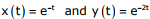which exist for t > 0, the convolution z(t) = x(t)* y(t) is
Explaination / Solution:Q5. Let the Laplace transform of a function F(t) which exists for t > 0 be F1(s) and the Laplace transform of its delayed version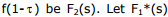be the complex conjugate of F1(s) with the Laplace variable set as s=σ + jw. If G(s) =, then the inverse Laplace transform of G(s) is
Explaination / Solution:Q6. A zero mean random signal is uniformly distributed between limits –a and +a and its mean square value is equal to its variance. Then the r.m.s value of the signal is
Explaination / Solution:Q7. The response h(t) of a linear time invariant system to an impulse δ(t) , under initially relaxed condition is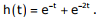The response of this system for a unit step input u(t) is
Explaination / Solution: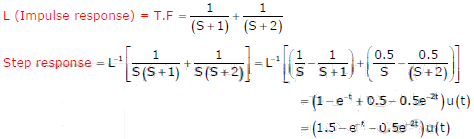Q8. Given that f(y) = | y | / y, and q is any non-zero real number, the value of | f(q) - f(-q) | is
Explaination / Solution: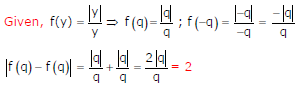Q9.
A periodic voltage waveform observed on an oscilloscope across a load is shown. A permanent magnet moving coil (PMMC) meter connected across the same load reads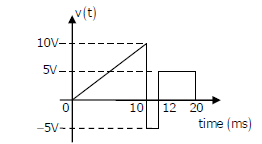Explaination / Solution:Q10. The state variable description of an LTI system is given by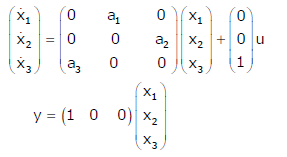where y is the output and u is the input. The system is controllable for

Explaination / Solution: